Welcome to Wall Street Prep! Use code at checkout for 15% off.# Debt Sizing in Project Finance

How gearing and DSCR impact debt sizing in project finance models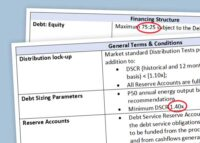## Debt sizing in project finance

Debt sizing refers to the project finance model mechanics for determining how much debt can be raised to support an infrastructure project.

The amount of debt that can be raised is defined in the debt term sheet and is usually expressed by a maximum gearing (leverage) ratio (e.g. maximum of 75% debt and 25% equity) and a minimum Debt Service Coverage Ratio (DSCR) (e.g. no less than 1.4x). The model then iterates (often using a debt sizing macro) to arrive at the implied debt size.

#### Get the Free Project Finance Model TemplateSubmitting...

## Introduction to Debt Sizing in Project Finance

Firstly, it’s important to set the scene. A term sheet might have something like this: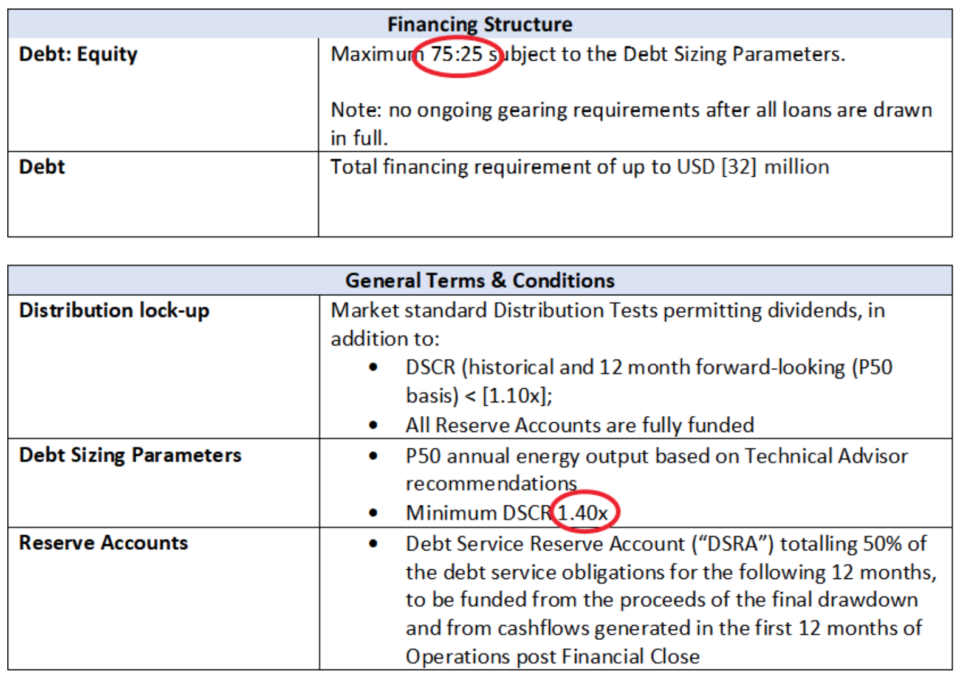This term sheet is for a renewables deal (you can tell from the “P50 energy output”). It gives us all the information we need for debt sizing – the gearing ratio of 75%, and the min DSCR of 1.40x (applied to a P50 revenue, in this case).

Let’s go through the 75% and the 1.40x separately.

## Maximum gearing ratio

Most people are familiar with this. We’re gearing the project, yes, but 75% of what? Outside of project finance, this is typically thought of as Loan To Cost (LTC).

The Cost part is the total funding amount, for example:

Project Finance Cost:

Construction costs

(+) interest during construction (IDC)

(+) financing fees (FF)

(+) other items (e.g. the DSRA initial funding amount).

## Minimum DSCR

In the term sheet above, at all points throughout the debt tenor, the DSCR must be greater than 1.40x. How can we rearrange the formula to calculate the debt size out of this?

Recalling our formula from our article on DSCR:

DSCR = CFADS / (Principal + Interest Payments)

Re-arranging the terms we get:

Principal + Interest (aka Debt Service) = CFADS/DSCR.

Rearranging again and summing these cash-flows over the debt tenor we get:

Principal Payments = CFADS / DSCR – Interest Payments

Now if we sum up all the principals, then we get back to what the maximum principal repayable is. Understand that we needed to run all the CFADS forecasts to arrive at this maximum debt size.

If you think about it, the maximum principal repayable, is really what your maximum debt size is. Because unpaid debt is a big no-no.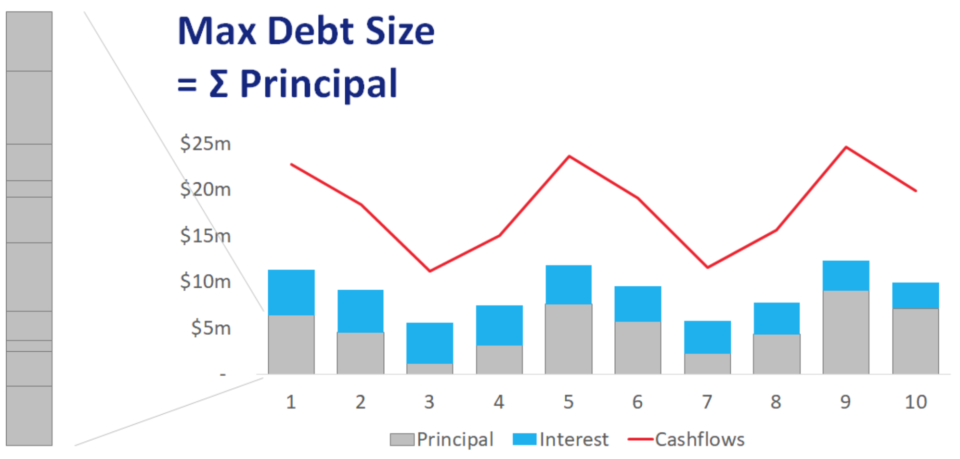The project finance model screenshot below shows the maximum principal repayment, and the opening balance.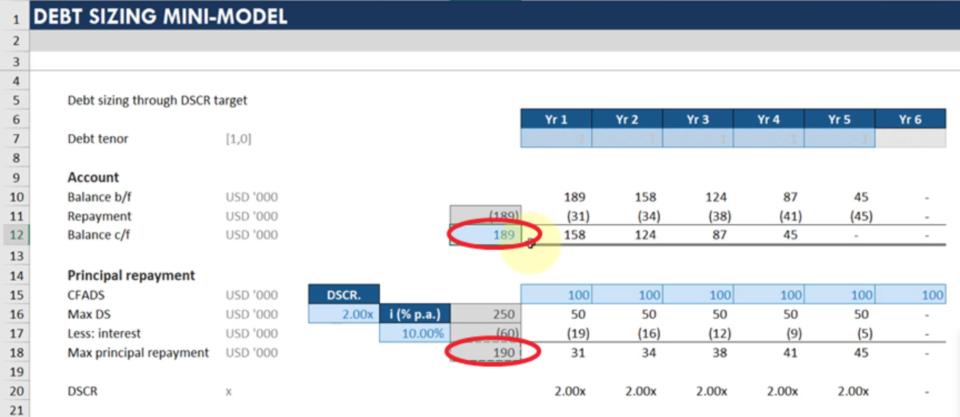Note that linking these would result in a circularity. Why? Following the chain of logic here: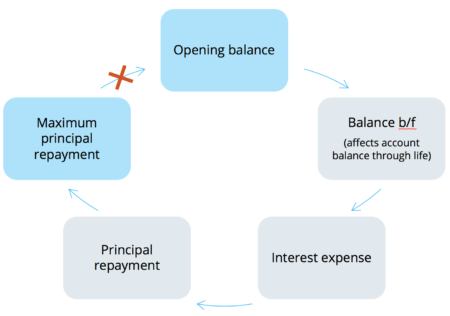For the gearing ratio debt calculation, each subsequent debt amount must take into account the construction costs & interest & fees generated off that debt, thereby increasing the funding amount, thereby increasing the debt size (to retain the 75% of funding met by debt).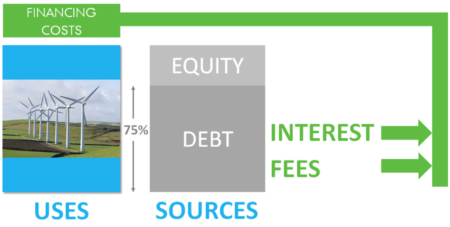Both of these calculations can be solved iteratively, and Excel has this functionality through the Iterative calculation feature. However this is not recommended at all – firstly because it will massively slow your model down – imagine instead of doing 1 calculation every time you press enter, it does 100… and secondly because the answer risks not converging (i.e. iterative process incomplete) or converging on the wrong solution. We remain in control of this by using a debt sizing macro.Step-by-Step Online Course

### The Ultimate Project Finance Modeling Package

Everything you need to build and interpret project finance models for a transaction. Learn project finance modeling, debt sizing mechanics, running upside/downside cases and more.

## Macros don’t break a circularity, they bridge it

At this point we need to restructure our models to break the circularity. This is basically breaking the circular chain – kind of like a circuit breaker would in an electric circuit. The way to do this is by using a Calculated and Applied logic: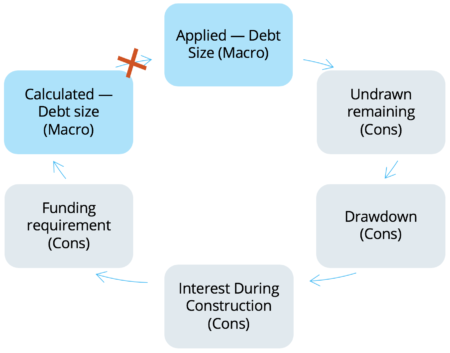• Calculated is where the debt feeds through from gearing calculations (e.g. 75% * funding required) and sculpting calculations (e.g. max principal).
• Applied feeds through the rest of the  model – e.g. restricting drawdowns in construction to the facility size etc
• They are not connected. You can connect them simply through copying the calculated lines and pasting them in the applied cells (try paste values!).

How this looks in a model is something like this: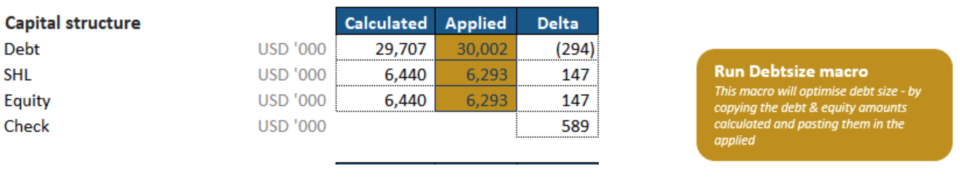## Debt sizing is an iterative process to converge on the solution

Every time the Calculated column is copied and pasted into the Applied column, the calculated column will change again. That is the nature of the circularity. The input depends on the output. Thus it requires a number of iterations to solve. How many? Could be as few as 5, could be a few hundred, depending on the calculation involved.

That should give you a good idea of how to think about debt sizing, for both gearing and DSCR in project finance. This still leaves us with a manual solution of copy and pasting values to bridge the divide between the Calculate and the Applied side. Macros automate this

### [Free Video]: Creating a Debt Sizing MacroBy Kyle Chaning Pearce
Kyle is a financial modeling instructor/practitioner with years of experience of project finance modeling training at Investment Banks, Funds, Project Developers, Big 4 Accountancy firms and more. Kyle was previously head of training EMEA at Mazars Global Infrastructure Finance, and trained professionals and students in project finance financial modeling around the world with project finance consulting firm Corality.
Inline Feedbacks